You are here:   ArielOrtiz.com > Programming Languages > Higher-Order Functions# Higher-Order Functions

## Objectives

During this activity, students should be able to:

• Write higher-order functions using the Clojure programming language.

This activity helps the student develop the following skills, values and attitudes: ability to analyze and synthesize, capacity for identifying and solving problems, and efficient use of computer systems.

## Activity Description

Individually, solve the following set of programming exercises using Clojure. Place all your functions in a namespace called `higherorder`.

1. The function `my-map-indexed` takes two arguments: a function f and a list lst. It returns a list consisting of the result of applying f to 0 and the first item of lst, followed by applying f to 1 and the second item in lst, and so on until lst is exhausted. Function f should accept 2 arguments: index and item. Do not use the predefined `map-indexed` function. Unit tests:
```(deftest test-my-map-indexed
(is (= () (my-map-indexed vector ())))
(is (= '([0 a] [1 b] [2 c] [3 d])
(my-map-indexed vector '(a b c d))))
(is (= '(10 4 -2 8 5 5 13)
(my-map-indexed + '(10 3 -4 5 1 0 7))))
(is (= '(0 1 -4 3 1 0 6)
(my-map-indexed min '(10 3 -4 5 1 0 7)))))```
2. The function `my-drop-while` takes two arguments: a function f and a list lst. It returns a list of items from lst dropping the initial items that evaluate to true when passed to f. Once a false value is encountered, the rest of the list is returned. Function f should accept one argument. Do not use the predefined `drop-while` function. Unit tests:
```(deftest test-my-drop-while
(is (= () (my-drop-while neg? ())))
(is (= '(0 1 2 3 4)
(my-drop-while
neg?
'(-10 -9 -8 -7 -6 -5 -4 -3 -2 -1 0 1 2 3 4))))
(is (= '(2 three 4 five)
(my-drop-while
symbol?
'(zero one 2 three 4 five))))
(is (= '(0 one 2 three 4 five)
(my-drop-while
symbol?
'(0 one 2 three 4 five)))))```
3. The bisection method is a root-finding algorithm which works by repeatedly dividing an interval in half and then selecting the subinterval in which the root exists.

Suppose we want to solve the equation f(x) = 0. Given two points a and b such that f(a) and f(b) have opposite signs, f must have at least one root in the interval [a, b] as long as f is continuous on this interval. The bisection method divides the interval in two by computing c = (a+b) / 2. There are now two possibilities: either f(a) and f(c) have opposite signs, or f(c) and f(b) have opposite signs. The bisection algorithm is then applied to the sub-interval where the sign change occurs.

Write the function `bisection`, that takes a, b, and f as arguments. It finds the corresponding root using the bisection method. The algorithm must stop when a value of c is found such that: abs(f(c)) < 1.0×10-15.

Unit tests:

```(deftest test-bisection
(is (= 3.0 (bisection 1 4 (fn [x] (* (- x 3) (+ x 4))))))
(is (= -4.0 (bisection -5 0 (fn [x] (* (- x 3) (+ x 4))))))
(is (= Math/PI (bisection 1 4 (fn [x] (Math/sin x)))))
(is (= (* 2 Math/PI) (bisection 5 10 (fn [x] (Math/sin x)))))
(is (= 1.618033988749895
(bisection 1 2 (fn [x] (- (* x x) x 1)))))
(is (= -0.6180339887498948
(bisection -10 1 (fn [x] (- (* x x) x 1))))))```

Use the `Math/abs` function to compute the absolute value of a number.

4. The derivate of a function f(x) with respect to variable x is defined as: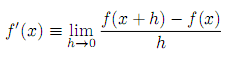Where f must be a continuous function. Write the function `deriv` that takes f and h as its arguments, and returns a new function that takes x as argument, and which represents the derivate of f given a certain value for h.

The unit tests verify the following derivates: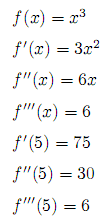Unit tests:

```(defn f [x] (* x x x))
(def df (deriv f 0.001))
(def ddf (deriv df 0.001))
(def dddf (deriv ddf 0.001))

(deftest test-deriv
(is (= 75 (Math/round (df 5))))
(is (= 30 (Math/round (ddf 5))))
(is (= 6 (Math/round (dddf 5)))))```
5. Simpson's rule is a method for numeric integration: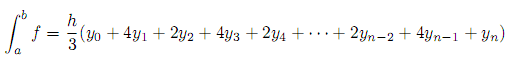Where n is an even positive integer (if you increment the value of n you get a better approximation), and h and yk are defined as follows: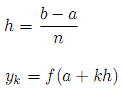Write the function `integral`, that takes as arguments a, b, n, and f. It returns the value of the integral, using Simpson's rule. The unit tests verify the following single and double integrals (with n = 10):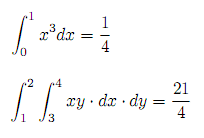Unit tests:

```(deftest test-integral
(is (= 1/4 (integral 0 1 10 (fn [x] (* x x x)))))
(is (= 21/4
(integral 1 2 10
(fn [x]
(integral 3 4 10
(fn [y]
(* x y))))))))```

## Deliverables

Deliver a single file called `higherorder.clj` containing your solutions and the unit tests. Please provide the following information:

IMPORTANT: The program source file must include at the top the author's personal information (name and student id) within comments. For example:

```
;;; ITESM CEM, September 14, 2014.
;;; Clojure Source File
;;; Activity: Higher-Order Functions
;;; Author: Steve Rogers, 1166611
.
. (The rest of the program goes here)
.```

Due date is Sunday, September 14.

## Evaluation

This activity will be evaluated using the following criteria:

 -10 The program doesn't contain within comments the author's personal information. The program contains syntax errors. The program was plagiarized. Depending on the amount of exercises that were solved correctly.